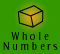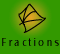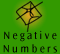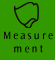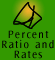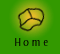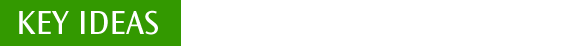| Conversions | Estimation |
| Indicating accuracy of measurements| Quick quiz |

Conversions

In order to be able to make and manipulate a range of measurements we need to be able to convert measurements into units which suit our situation. We can measure an object using quite small units. This permits the measure to be more accurate. If we want to, we can convert our measurement into a more manageable unit when we have finished our calculations.

The following diagram shows how we can convert length measurements into smaller or larger units. For example, to convert a measurement in metres to centimetres, multiply the number of metres by 100. To convert a measurement in centimetres to metres, divide the number of centimetres by 100.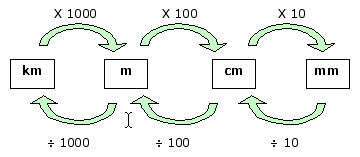Example 1: Full explanation of length measurement conversion.
According to a local map the shortest route to the gym is about 3080 metres, how many kilometres is this?

 Thinking There are 1000m in one kilometre. To go from metres (a smaller unit) to kilometres (a bigger unit), I need to divide by 1000 to make the number of units smaller. 3080 divided by 1000 is 3.080, so the distance to the gym is 3.08 km.

Some other measurement conversion examples are shown below.

Example 2: Full explanation of volume measurement conversion.
How many litres are in 2 Megalitres?

Example 3: Full explanation of area measurement conversion.
How many square metres are in 6 hectares?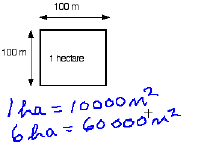Click the image to view the movie Do you notice anything wrong with this movie? The speaker in this movie refers to the units of the block as 'metres squared'. The correct expression is 'square metres'.

Although builders would measure the length of a swimming pool or the height of a wall in millimetres, we would probably use metres.

Example 4: Measurement conversion.
A national swimming meet is proposed to be held at the local swimming pool. To ensure that the pool is appropriate for the competition, an engineer has to check its exact length. At a site inspection she measured the length of the swimming pool to be 50130 mm. How many metres is this? If the pool must be exactly 50 m long, can it be used for the competition?

 Working Out Thinking 1000 mm = 1 m 50130 mm = ? m 50130 mm = 50130/1000 m So, 50130 mm = 50.130 m The pool is 13 centimetres too long to use for the competition. I know that there are 1000 millimetres in a metre because 'milli' means one thousand. To convert from a smaller unit to a larger unit, I must divide. So in order to convert millimetres to metres I need to divide my measurement by one thousand. 50,130 mm divided by 1000 equals 50.130 m. 50.130 m is 50 m and 13 cm. Therefore the pool is 13 cm too long.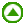Estimation

We use measurement regularly in our daily lives. Often, an estimate may be sufficient, but in other cases accurate measurement is very important. Engineers and builders need to be as exact as possible in their measurements when constructing buildings. Scientists need to be extremely accurate when mixing minute amounts of chemicals.

Depending on our situation, we make choices about how we make measurements. We might consider the following things:

- smaller units of measure allow us to measure more accurately

- an estimation using informal units may be sufficient for our needs

- having an idea of benchmarks helps us to make estimations.

Informal or Non-standard units
When an estimation is sufficient for our needs, we often use informal measurements of length and then approximate these informal units to standard units. These informal units may include a palm's width as we know that a palm is approximately 10 cm wide or a long stride which may be about 1 metre long.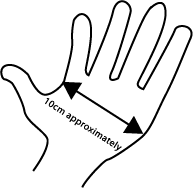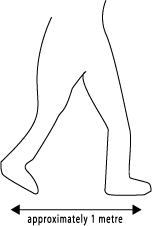Example 5: Measuring with informal units.
Ivan decides to count how many strides it takes to walk the perimeter of a reservoir. He estimates that his strides are about 80 centimetres long. If he makes 125 strides to complete the walk, how many metres is this?

 Working Out Thinking 1 stride = 80 cm 125 strides = 125 x 80 cm 125 strides = 10000 cm 100 cm = 1 m 10000 cm = 100 m The reservoir has a perimeter of approximately 100 metres. I know 1 stride is approximately 80 cm in length, therefore 125 strides, which is the perimeter, would be 125 x 80 cm. 125 x 80 = 10000 I now need to convert centimetres into metres. There are 100 cm in 1 m. I am converting from a smaller unit to a larger unit so I must divide. So in order to convert my measurement in cm into m I need to divide by 100.

Indicating accuracy of measurements

Is a measurement of 2km the same as a measurement of 2.000 km? Certainly the decimal number 2 has exactly the same value as the number 2.000.

With measurement, however, these two measures have a different meaning. The number of places indicates the accuracy of the measurement. If a distance is given as 2 km, this means that it is closer to 2 km than to 1 km or 3 km. It is somewhere between 1.5 and 2.5 km. The measurement of 2.000 km indicates a much more precise measurement. It is nearer to 2.001 km than to 1.999 km. Writing the measurement as 2.000 km indicates that the distance has been measured to the nearest metre. If the distance was given as 2.0000 km, it would indicate that it has been measured to the nearest one tenth of a metre.

A temperature given as 23.6 degrees indicates that it has been measured to the nearest tenth of a degree. If the temperature is given as 23.60 degrees, this indicates it has been measured more accurately (to the nearest one hundredth of a degree). If it is given as 23 degrees, it has been measured less accurately.

Be sure to consider carefully the number of decimal places you use when reporting a measurement. These significant figures are very important in scientific and engineering work.Quick quiz

 1. Convert 1400 metres into kilometres. 2. Convert 2.637 kilograms to grams. 3. Convert 4.5 kilolitres into litres. 4. Estimate the length of your stride in centimetres and calculate the approximate distance in metres it is from your house to the nearest corner.

5. Joshua estimates that the length of his desk is about 15.5 times the width of his palm. He measures his palm and finds it is 9 cm wide. What is the approximate length of his desk?

6. Naomi measures a bookcase and finds it is 160.5cm high. Matthew measures the same bookcase and finds it is 160.50cm high. Comment on their measurements.

7. Complete the following statements.

(a) 385 centimetres = ____________ metres

(b) 9.35 kilograms = _____________ grams

(c) 49 millilitres = _______________ litres

(d) 0.054km = __________________ centimetres

(e) 8647km = ___________________ metres

8. I have 3m 65cm of alfoil left on my roll. How many centimetres are left?

9. Martin’s stride is 80 cm. How many metres does he walk if he takes 60 strides?

10. How many 25g packets of rice can be made from 1 kg?

11. How many seconds are there from 9.00pm Sunday to 7.00am the next day,Monday?

12. Which is larger ­ 3250 ml or 0.325 litres? Give a reason for your answer.